##### Basic Math & Pre-Algebra All-in-One For Dummies (+ Chapter Quizzes Online)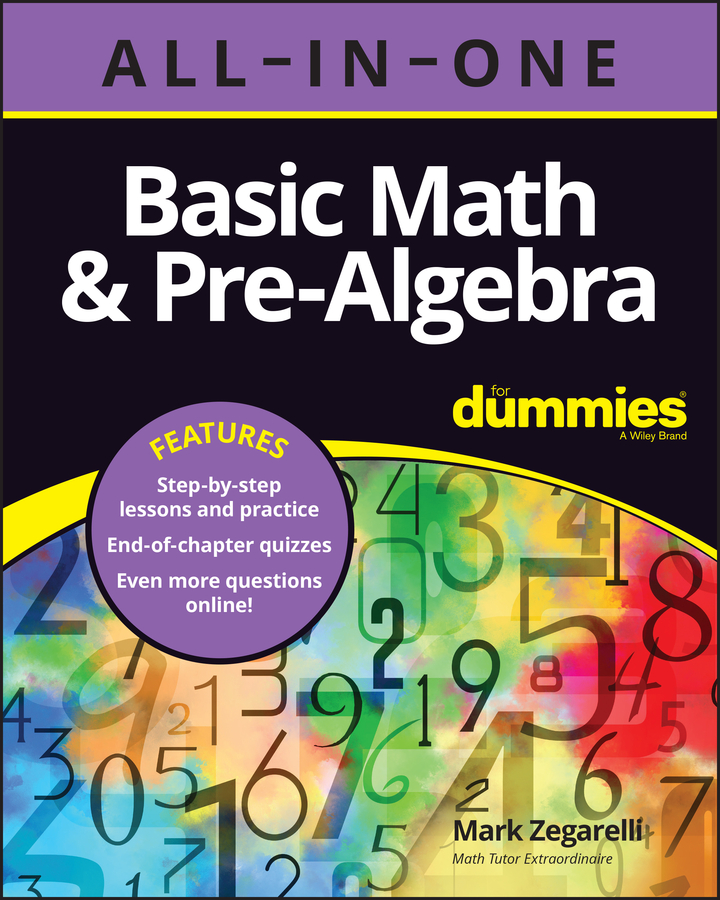When you know how to do long division, figuring out how to divide decimals is easy. However, unlike dividing whole numbers, with decimals, you have to add trailing zeros in the dividend and you also have to complete the problem without remainders.

The main difference comes at the beginning, before you start dividing.

## Do a simple division problem

Here’s how to do a simple division problem:

1. Turn the divisor (the number you’re dividing by) into a whole number by moving the decimal point all the way to the right; at the same time, move the decimal point in the dividend (the number you’re dividing) the same number of places to the right.

For example, suppose you want to divide 10.274 by 0.11. Write the problem as usual: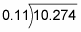Turn 0.11 into a whole number by moving the decimal point in 0.11 two places to the right, giving you 11. At the same time, move the decimal point in 10.274 two places to the right, giving you 1,027.4: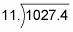2. Place a decimal point in the quotient (the answer) directly above where the decimal point now appears in the dividend.

Here’s what this step looks like: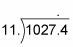3. Divide as usual, being careful to line up the quotient properly so that the decimal point falls into place.

To start out, notice that 11 is too large to go into either 1 or 10. However, 11 does go into 102 (9 times). So write the first digit of the quotient just above the 2 and continue: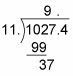Bring down the next number, 7. This time, 11 goes into 37 three times. The important thing is to place the next digit in the answer just above the 7: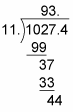Bring down the next number, 4. Now, 11 goes into 44 four times. Again, be careful to place the next digit in the quotient just above the 4, and complete the division: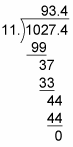So the answer is 93.4. As you can see, as long as you’re careful when placing the decimal point and the digits, the correct answer appears with the decimal point in the right position.

## Add trailing zeros in the dividend

Sometimes, you may have to add one or more trailing zeros to the dividend. You can add as many trailing zeros as you like to a decimal without changing its value. For example, suppose you want to divide 67.8 by 0.333: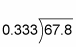1. Change 0.333 into a whole number by moving the decimal point three places to the right; at the same time, move the decimal point in 67.8 three places to the right: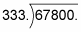In this case, when you move the decimal point in 67.8, you run out of room, so you have to add a couple of zeros to the dividend. This step is perfectly valid, and you need to do this whenever the divisor has more decimal places than the dividend.

2. Place the decimal point in the quotient directly above where it appears in the dividend: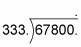3. Divide as usual, being careful to line up the numbers in the quotient correctly. This time, 333 doesn’t go into 6 or 67, but it does go into 678 (two times). So place the first digit of the quotient directly above the 8: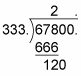This example jumps forward in the division to the place where you bring down the first 0. At this point, 333 doesn’t go into 120, so you need to put a 0 above the first 0 in 67,800 and bring down the second 0. Now, 333 does go into 1,200, so place the next digit in the answer (3) over the second 0: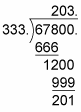This time, the division doesn’t work out evenly. If this were a problem with whole numbers, you’d finish by writing down a remainder of 201. But decimals are a different story. The next section explains why, with decimals, the show must go on.

## Complete decimal division

When you’re dividing whole numbers, you can complete the problem simply by writing down the remainder. But remainders are never allowed in decimal division.

A common way to complete a problem in decimal division is to round off the answer. In most cases, you’ll be instructed to round your answer to the nearest whole number or to one or two decimal places.

To complete a decimal division problem by rounding it off, you need to add at least one trailing zero to the dividend. To round a decimal to

• a whole number, add one trailing zero

• one decimal place, add two trailing zeros

• two decimal places, add three trailing zeros

Here’s what the problem looks like with a trailing zero attached: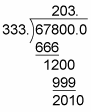Attaching a trailing zero doesn’t change a decimal, but it does allow you to bring down one more number, changing 201 into 2,010. Now you can divide 333 into 2,010: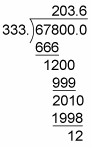At this point, you can round the answer to the nearest whole number, 204.